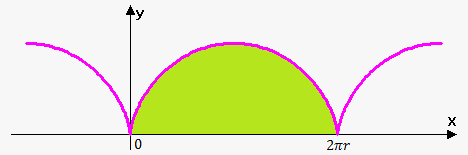# Area Enclosed by Parametric Curves

We know that area under the curve y=F(x) is A=int_a^b F(x)dx where f(x)>=0.

If curve is given by parametric equations x=f(t) and y=g(t) then using substitution rule with x=f(t) we have that dx=f'(t)dt and since x is changing from a to b then t is changing from alpha=f^(-1)(a) to beta=f^(-1)(b). It is not always a case, sometimes t is changing from beta to alpha.

So, A=int_a^bF(x)dx=int_alpha^beta F(f(t))f'(t)dt=int_alpha^beta g(t)f'(t)dt.

Area Enclosed by Parametric Curves: A=int_(alpha)^(beta)g(t)f'(t)dt (or int_beta^alpha g(t)f'(t)dt).

Example. Find the area under one arch of cycloid. x=r(t-sin(t)), y=r(1-cos(t)).One arch of the cycloid is given by 0<=t<=2pi.

We have that x_t'=r(1-cos(t)).

Therefore, A=int_0^(2pi)r(1-cos(t))r(1-cos(t))dt=

=r^2 int_0^(2pi)(1-cos(t))^2dt=r^2 int_0^(2pi) (1-2cos(t)+cos^2(t))dt.

Now, we need to use double angle formula and integral becomes:

Ar^2 int_0^(2pi)(1-2cos(t)+1/2(1+cos(2t)))dt=r^2 int_0^(2pi) (3/2 -2cos(t)+1/2cos(2t))dt=

=r^2(3/2 t-2sin(t)+1/4sin(2t))|_0^(2pi)=

=r^2((3/2 2pi-2sin(2pi)+1/4sin(2* 2pi))-(3/2 *0-2sin(0)+1/4sin(2*0)))=

=r^2((3pi-2*0+1/4*0)-(0-2*0+1/4*0))=3pir^2.